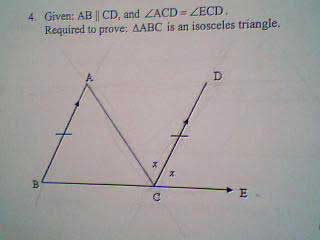SEARCH HOMEMath Central Quandaries & QueriesQuestion from Lisa, a student: I am stuck on this problem... If AB = CD (parellel line), andLias continued.

I was able to take a pic with my web cam so you'll get full details on the pic since I don't know how to draw you the pic.Hi Lisa.

1. Corresponding angles formed by a transverse line crossing two parallel lines are congruent, therefore angle CAB equals angle ACD and that's x in your diagram.

2. For similar reasons, angle ABC equals angle DCE and that's also x.

The final step is connected to another problem you sent us.

Question from Lisa, a student:

This is a fill in the blanks that I just do not understand.

The (blank) angle of a triangle is equal to the sum of the (blank) opposite angles.

Can you formalize this and complete the proof?

The
Stephen and Penny

PS: Your web camera really helped!Math Central is supported by the University of Regina and The Pacific Institute for the Mathematical Sciences.